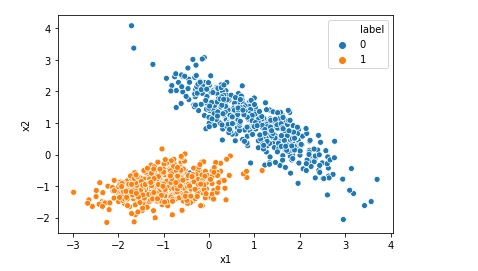# Difference between Logistic Regression and Support Vector Machine SVM

Both Logistic Regression and Support Vector Machine or SVM can be used for classification however there is some fundamental difference between them.

Lets understand those differences when we are using Support Vector Machine as a classifier.

1. Logistic Regression gives probabilities as output that can be interpreted as confidence in the classification decision however Support Vector machine does not provide probabilistic output as it is a non-probabilistic model.
2. If number of features is small compared to number of rows in the dataset then Support Vector Machine is more appropriate than Logistic Regression.
3. If number of features is more compared to number of rows in the training dataset, we should prefer logistic regression and if you need to go with SVM in this case, choose SVM with a linear kernel.
4. In case of Logistic Regression, farther the data point lies from the separating line on the correct side, the better confidence factor the data point will get. However in case of Support Vector Machine, if a point is not a support vector it does not makes any difference, how far the data point is from the separating line, of course on the correct side.

The above four are the main difference between these two classification algorithm.

Cheers !!